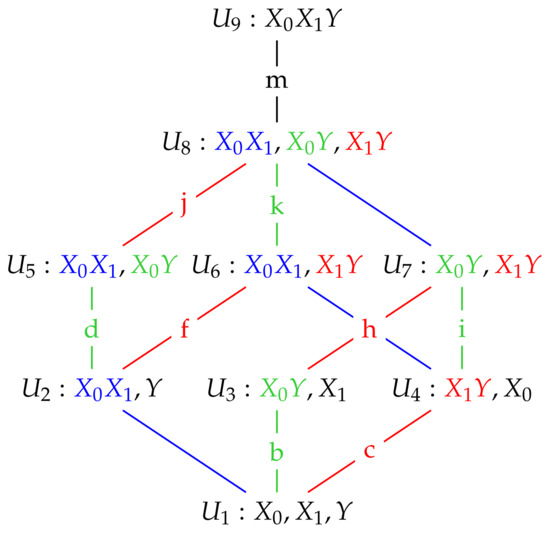Previous Article in Journal
2D Tsallis Entropy for Image Segmentation Based on Modified Chaotic Bat Algorithm
Article

Exact Partial Information Decompositions for Gaussian Systems Based on Dependency Constraints

1
Department of Statistics, University of Glasgow, Glasgow G12 8QQ, UK
2
Institute of Neuroscience and Psychology, University of Glasgow, Glasgow G12 8QQ, UK
*
Author to whom correspondence should be addressed.
Entropy 2018, 20(4), 240; https://doi.org/10.3390/e20040240
Received: 9 March 2018 / Revised: 26 March 2018 / Accepted: 27 March 2018 / Published: 30 March 2018
The Partial Information Decomposition, introduced by Williams P. L. et al. (2010), provides a theoretical framework to characterize and quantify the structure of multivariate information sharing. A new method ( $I dep$ ) has recently been proposed by James R. G. et al. (2017) for computing a two-predictor partial information decomposition over discrete spaces. A lattice of maximum entropy probability models is constructed based on marginal dependency constraints, and the unique information that a particular predictor has about the target is defined as the minimum increase in joint predictor-target mutual information when that particular predictor-target marginal dependency is constrained. Here, we apply the $I dep$ approach to Gaussian systems, for which the marginally constrained maximum entropy models are Gaussian graphical models. Closed form solutions for the $I dep$ PID are derived for both univariate and multivariate Gaussian systems. Numerical and graphical illustrations are provided, together with practical and theoretical comparisons of the $I dep$ PID with the minimum mutual information partial information decomposition ( $I mmi$ ), which was discussed by Barrett A. B. (2015). The results obtained using $I dep$ appear to be more intuitive than those given with other methods, such as $I mmi$ , in which the redundant and unique information components are constrained to depend only on the predictor-target marginal distributions. In particular, it is proved that the $I mmi$ method generally produces larger estimates of redundancy and synergy than does the $I dep$ method. In discussion of the practical examples, the PIDs are complemented by the use of tests of deviance for the comparison of Gaussian graphical models. View Full-Text
Show FiguresFigure 1

MDPI and ACS Style

Kay, J.W.; Ince, R.A.A. Exact Partial Information Decompositions for Gaussian Systems Based on Dependency Constraints. Entropy 2018, 20, 240. https://doi.org/10.3390/e20040240

AMA Style

Kay JW, Ince RAA. Exact Partial Information Decompositions for Gaussian Systems Based on Dependency Constraints. Entropy. 2018; 20(4):240. https://doi.org/10.3390/e20040240

Chicago/Turabian Style

Kay, Jim W., and Robin A.A. Ince. 2018. "Exact Partial Information Decompositions for Gaussian Systems Based on Dependency Constraints" Entropy 20, no. 4: 240. https://doi.org/10.3390/e20040240

Find Other Styles
Note that from the first issue of 2016, MDPI journals use article numbers instead of page numbers. See further details here.

1